CGAL 5.6 - dD Triangulations
TriangulationDataStructure Concept Reference

## Definition

The TriangulationDataStructure concept describes objects responsible for storing and maintaining the combinatorial part of a $$d$$-dimensional pure simplicial complex that has the topology of the $$d$$-dimensional sphere $$\mathbb{S}^d$$ with $$d\in[-2,D]$$. Since the simplicial $$d$$-complex is pure, all faces are sub-faces of some $$d$$-simplex. And since it has the topology of the sphere $$\mathbb{S}^d$$, it is manifold, thus any $$d-1$$-face belongs to exactly two $$d$$-dimensional full cells.

The concept TriangulationDataStructure includes two sub-concepts TriangulationDataStructure::Vertex and TriangulationDataStructure::FullCell.

Possible values for the current dimension $$d$$ include

-2
This corresponds to the non-existence of any object in the triangulation.
-1

This corresponds to a single vertex and a single full cell, which is also the unique vertex and the unique full cell in the TriangulationDataStructure. In a geometric realization of the TriangulationDataStructure (e.g., in a Triangulation<TriangulationTraits_, TriangulationDataStructure_> or a Delaunay_triangulation<DelaunayTriangulationTraits_, TriangulationDataStructure_>), this vertex corresponds to the vertex at infinity.

0

This corresponds to two vertices, each incident to one $$0$$-face; the two full cells being neighbors of each other. This is the unique triangulation of the $$0$$-sphere.

$$d>0$$
This corresponds to a triangulation of the sphere $$\mathbb{S}^d$$.

An $$i$$-simplex is a simplex with $$i+1$$ vertices. An $$i$$-simplex $$\sigma$$ is incident to a $$j$$-simplex $$\sigma'$$, $$j<i$$, if and only if $$\sigma'$$ is a proper face of $$\sigma$$.

We call a $$0$$-simplex a vertex, a $$(d-1)$$-simplex a facet and a $$d$$-simplex a full cell. A face can have any dimension. Two full cells are neighbors if they share a facet. Two faces are incident if one is included in the other.

Input/Output

The information stored in the iostream is:

• the current dimension (which must be smaller than or equal to tds.maximal_dimension()),
• the number of vertices,
• for each vertex the information of that vertex,
• the number of full cells,
• for each full cell the indices of its vertices and extra information for that full cell,
• for each full cell the indices of its neighbors.

The indices of vertices and full cells correspond to the order in the file; the user cannot control it. The classes Vertex and Full_cell have to provide the relevant I/O operators (possibly empty).

Has Models:
CGAL::Triangulation_data_structure<Dimensionality, TriangulationDSVertex_, TriangulationDSFullCell_>
TriangulationDataStructure::Vertex
TriangulationDataStructure::FullCell
TriangulationDSVertex
TriangulationDSFullCell
TriangulationDSFace

## Concepts

conceptFullCell
The concept TriangulationDataStructure::FullCell describes the type used by a TriangulationDataStructure to store the full cells. More...

conceptVertex
The concept TriangulationDataStructure::Vertex describes the type used by a TriangulationDataStructure to store the vertices. More...

## Types

typedef unspecified_type Vertex
The vertex type, requirements for this type are described in the concept TriangulationDataStructure::Vertex.

typedef unspecified_type Full_cell
The full cell type, requirements for this type are described in the concept TriangulationDataStructure::FullCell.

typedef unspecified_type Full_cell_data
A model of the concept FullCellData.

typedef unspecified_type Facet
The facet type, for describing faces of the triangulation with codimension 1. More...

typedef unspecified_type Face
The face type, which must be a model of the concept TriangulationDSFace.

## Handles

Vertices and full cells are manipulated via handles.

Handles support the usual two dereference operators and operator->.

typedef unspecified_type Vertex_handle
Handle to a Vertex.

typedef unspecified_type Vertex_const_handle
Const handle to a Vertex.

typedef unspecified_type Full_cell_handle
Handle to a Full_cell.

typedef unspecified_type Full_cell_const_handle
Const handle to a Full_cell.

## Iterators

Vertices, facets and full cells can be iterated over using iterators.

Iterators support the usual two dereference operators and operator->.

typedef unspecified_type Vertex_iterator
Iterator over the list of vertices.

typedef unspecified_type Full_cell_iterator
Iterator over the list of full cells.

typedef unspecified_type Facet_iterator
Iterator over the facets of the complex.

typedef unspecified_type size_type
Size type (an unsigned integral type).

typedef unspecified_type difference_type
Difference type (a signed integral type).

## Creation

TriangulationDataStructure (int dim=0)
Creates an instance tds of type TriangulationDataStructure. More...

## Queries

int maximal_dimension () const
Returns the maximal dimension of the full dimensional cells that can be stored in the triangulation tds. More...

int current_dimension () const
Returns the dimension of the full dimensional cells stored in the triangulation. More...

bool empty () const
Returns true if the triangulation contains nothing, returns false otherwise.

size_type number_of_vertices () const
Returns the number of vertices in the triangulation.

size_type number_of_full_cells () const
Returns the number of full cells in the triangulation.

bool is_vertex (const Vertex_handle &v) const
Tests whether v is a vertex of the triangulation.

bool is_full_cell (const Full_cell_handle &c) const
Tests whether c is a full cell of the triangulation.

template<typename TraversalPredicate , typename OutputIterator >
Facet gather_full_cells (Full_cell_handle start, TraversalPredicate &tp, OutputIterator &out) const
This function computes (gathers) a connected set of full cells satisfying a common criterion. More...

template<typename OutputIterator >
OutputIterator incident_full_cells (Vertex_handle v, OutputIterator out) const
Inserts in out all the full cells that are incident to the vertex v, i.e., the full cells that have the Vertex v as a vertex. More...

template<typename OutputIterator >
OutputIterator incident_full_cells (const Face &f, OutputIterator out) const
Inserts in out all the full cells that are incident to the face f, i.e., the full cells that have the Face f as a subface. More...

template<typename OutputIterator >
OutputIterator star (const Face &f, OutputIterator out) const
Inserts in out all the full cells that share at least one vertex with the Face f. More...

template<typename OutputIterator >
OutputIterator incident_faces (Vertex_handle v, const int dim, OutputIterator out)
Constructs all the Faces of dimension dim incident to Vertex v and inserts them in the OutputIterator out. More...

## Accessing the Vertices

Vertex_handle vertex (Full_cell_handle c, const int i) const
Returns a handle to the i-th Vertex of the Full_cell c. More...

int mirror_index (Full_cell_handle c, int i) const
Returns the index of c as a neighbor of its i-th neighbor. More...

Vertex_handle mirror_vertex (Full_cell_handle c, int i) const
Returns the vertex of the i-th neighbor of c that is not vertex of c. More...

Vertex_iterator vertices_begin ()
Iterator to the first vertex of tds. More...

Vertex_iterator vertices_end ()
Iterator referring beyond the last vertex of tds.

## Accessing the Full Cells

Full_cell_handle full_cell (Vertex_handle v) const
Returns a full cell incident to Vertex v. More...

Full_cell_handle neighbor (Full_cell_handle c, int i) const
Returns a Full_cell_handle pointing to the Full_cell opposite to the i-th vertex of c. More...

Full_cell_iterator full_cells_begin ()
Iterator to the first full cell of tds. More...

Full_cell_iterator full_cells_end ()
Iterator referring beyond the last full cell of tds.

## Faces and Facets

Facet_iterator facets_begin ()
Iterator to the first facet of the triangulation.

Facet_iterator facets_end ()
Iterator referring beyond the last facet of the triangulation.

Full_cell_handle full_cell (const Facet &f) const
Returns a full cell containing the facet f

int index_of_covertex (const Facet &f) const
Returns the index of vertex of the full cell c=tds.full_cell(f) which does not belong to c.

## Vertex Insertion

Vertex_handle insert_in_full_cell (Full_cell_handle c)
Inserts a new vertex v in the full cell c and returns a handle to it. More...

Vertex_handle insert_in_face (const Face &f)
Inserts a vertex in the triangulation data structure by subdividing the Face f. More...

Vertex_handle insert_in_facet (const Facet &ft)
Inserts a vertex in the triangulation data structure by subdividing the Facet ft. More...

template<class ForwardIterator >
Vertex_handle insert_in_hole (ForwardIterator start, ForwardIterator end, Facet f)
This is an advanced function. More...

template<class ForwardIterator , class OutputIterator >
Vertex_handle insert_in_hole (ForwardIterator start, ForwardIterator end, Facet f, OutputIterator out)
Same as above, but handles to the new full cells are appended to the out output iterator.

Vertex_handle insert_increase_dimension (Vertex_handle star)
Transforms a triangulation of the sphere $$\mathbb{S}^d$$ into the triangulation of the sphere $$\mathbb{S}^{d+1}$$ by adding a new vertex v. More...

Full_cell_handle new_full_cell ()
Adds a new full cell to tds and returns a handle to it. More...

Vertex_handle new_vertex ()
Adds a new vertex to tds and returns a handle to it. More...

void associate_vertex_with_full_cell (Full_cell_handle c, int i, Vertex_handle v)
Sets the i-th vertex of c to v and, if v != Vertex_handle(), sets c as the incident full cell of v.

void set_neighbors (Full_cell_handle ci, int i, Full_cell_handle cj, int j)
Sets the neighbor opposite to vertex i of Full_cell ci to cj. More...

void set_current_dimension (int d)
Forces the current dimension of the complex to d. More...

## Vertex Removal

void clear ()
Reinitializes tds to the empty complex.

Vertex_handle collapse_face (const Face &f)
Contracts the Face f to a single vertex. More...

void remove_decrease_dimension (Vertex_handle v, Vertex_handle star)
This method does exactly the opposite of insert_increase_dimension(): v is removed, full cells not containing star are removed, full cells containing star but not v lose vertex star, full cells containing star and v lose vertex v (see Figure Figure 48.5). More...

void delete_vertex (Vertex_handle v)
This is an advanced function. More...

void delete_full_cell (Full_cell_handle c)
This is an advanced function. More...

template<typename ForwardIterator >
void delete_full_cells (ForwardIterator start, ForwardIterator end)
This is an advanced function. More...

## Validity Check

bool is_valid (bool verbose=false) const
This is a function for debugging purpose. More...

std::istream & operator>> (std::istream &is, TriangulationDataStructure &tds)
Reads a combinatorial triangulation from is and assigns it to tds. More...

std::ostream & operator<< (std::ostream &os, const TriangulationDataStructure &tds)
Writes tds into the output stream os.

## ◆ Facet

The facet type, for describing faces of the triangulation with codimension 1.

The constructor Facet(c,i) constructs a Facet representing the facet of full cell c opposite to its i-th vertex. Its dimension is current_dimension()-1.

## ◆ TriangulationDataStructure()

 TriangulationDataStructure::TriangulationDataStructure ( int dim = 0 )

Creates an instance tds of type TriangulationDataStructure.

The maximal dimension of its full cells is dim and tds is initialized to the empty triangulation. Thus, tds.current_dimension() equals -2. The parameter dim can be ignored by the constructor if it is already known at compile-time. Otherwise, the following precondition holds:

Precondition
dim>0.

## ◆ collapse_face()

 Vertex_handle TriangulationDataStructure::collapse_face ( const Face & f )

Contracts the Face f to a single vertex.

Returns a handle to that vertex (see Figure Figure 48.1).

Precondition
The boundary of the full cells incident to f is a topological sphere of dimension tds.current_dimension()-1).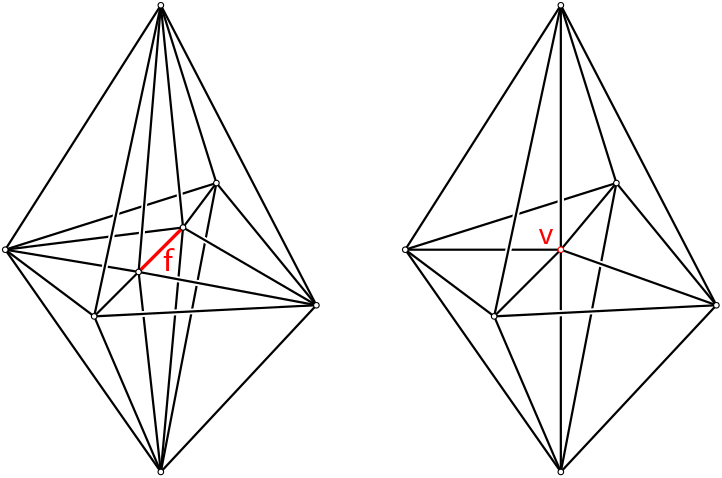Figure 48.1 Collapsing an edge in dimension $$d=3$$, v is returned.

## ◆ current_dimension()

 int TriangulationDataStructure::current_dimension ( ) const

Returns the dimension of the full dimensional cells stored in the triangulation.

It holds that tds.current_dimension()=-2 if and only if tds.empty() is true.

Postcondition
the returned value d satisfies $$-2\leq d \leq$$tds.maximal_dimension().

## ◆ delete_full_cell()

 void TriangulationDataStructure::delete_full_cell ( Full_cell_handle c )

Remove the full cell c from the triangulation.

Precondition
c is a full cell of tds.

## ◆ delete_full_cells()

template<typename ForwardIterator >
 void TriangulationDataStructure::delete_full_cells ( ForwardIterator start, ForwardIterator end )

Calls delete_full_cell over an iterator range of value type Full_cell_handle.

## ◆ delete_vertex()

 void TriangulationDataStructure::delete_vertex ( Vertex_handle v )

Remove the vertex v from the triangulation.

Precondition
v is a vertex of tds.

## ◆ full_cell()

 Full_cell_handle TriangulationDataStructure::full_cell ( Vertex_handle v ) const

Returns a full cell incident to Vertex v.

Note that this full cell is not unique (v is typically incident to more than one full cell).

Precondition
v != Vertex_handle

## ◆ full_cells_begin()

 Full_cell_iterator TriangulationDataStructure::full_cells_begin ( )

Iterator to the first full cell of tds.

User has no control on the order.

## ◆ gather_full_cells()

template<typename TraversalPredicate , typename OutputIterator >
 Facet TriangulationDataStructure::gather_full_cells ( Full_cell_handle start, TraversalPredicate & tp, OutputIterator & out ) const

This function computes (gathers) a connected set of full cells satisfying a common criterion.

Call them good full cells. It is assumed that the argument start is a good full cell. The full cells are then recursively explored by examining if, from a given good full cell, its adjacent full cells are also good.

The argument tp is a predicate, i.e. a function or a functor providing operator(), that takes as argument a Facet whose Full_cell is good. The predicate must return true if the traversal of that Facet leads to a good full cell.

All the good full cells are output into the last argument out.

Returns a facet on the boundary of the set of cells.

Precondition
start != Full_cell_handle() and start is a good cell.

## ◆ incident_faces()

template<typename OutputIterator >
 OutputIterator TriangulationDataStructure::incident_faces ( Vertex_handle v, const int dim, OutputIterator out )

Constructs all the Faces of dimension dim incident to Vertex v and inserts them in the OutputIterator out.

If dim >= tds.current_dimension(), then no Face is constructed.

Precondition
0 < dim and v!=Vertex_handle().

## ◆ incident_full_cells() [1/2]

template<typename OutputIterator >
 OutputIterator TriangulationDataStructure::incident_full_cells ( Vertex_handle v, OutputIterator out ) const

Inserts in out all the full cells that are incident to the vertex v, i.e., the full cells that have the Vertex v as a vertex.

Returns the output iterator.

Precondition
v!=Vertex_handle().

## ◆ incident_full_cells() [2/2]

template<typename OutputIterator >
 OutputIterator TriangulationDataStructure::incident_full_cells ( const Face & f, OutputIterator out ) const

Inserts in out all the full cells that are incident to the face f, i.e., the full cells that have the Face f as a subface.

Returns the output iterator.

Precondition
f.full_cell()!=Full_cell_handle().

## ◆ insert_in_face()

 Vertex_handle TriangulationDataStructure::insert_in_face ( const Face & f )

Inserts a vertex in the triangulation data structure by subdividing the Face f.

Returns a handle to the newly created Vertex.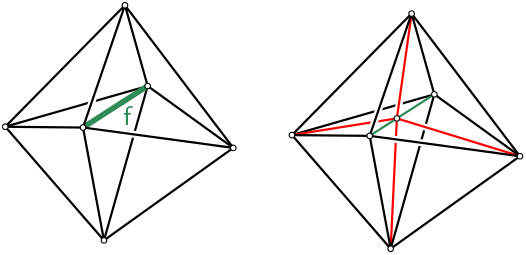Figure 48.2 Insertion in face, $$d=3$$

## ◆ insert_in_facet()

 Vertex_handle TriangulationDataStructure::insert_in_facet ( const Facet & ft )

Inserts a vertex in the triangulation data structure by subdividing the Facet ft.

Returns a handle to the newly created Vertex.

## ◆ insert_in_full_cell()

 Vertex_handle TriangulationDataStructure::insert_in_full_cell ( Full_cell_handle c )

Inserts a new vertex v in the full cell c and returns a handle to it.

The full cell c is subdivided into tds.current_dimension()+1 full cells which share the vertex v.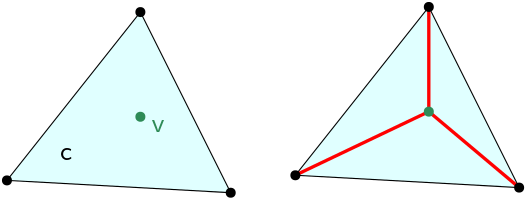Figure 48.3 Insertion in a full cell, $$d=2$$

Precondition
Current dimension is positive and c is a full cell of tds.

## ◆ insert_in_hole()

template<class ForwardIterator >
 Vertex_handle TriangulationDataStructure::insert_in_hole ( ForwardIterator start, ForwardIterator end, Facet f )

Removes the full cells in the range $$C=$$[s, e), inserts a vertex at position p and fills the hole by connecting each face of the boundary to p. A Vertex_handle to the new Vertex is returned. The facet ft must lie on the boundary of $$C$$ and its defining full cell, tr.full_cell(ft) must lie inside $$C$$. Handles to the newly created full cells are output in the out output iterator.

Precondition
c belongs to $$C$$ and c->neighbor(i) does not, with f=(c,i). $$H$$, the union of full cells in $$C$$, is simply connected and its boundary $$\partial H$$ is a combinatorial triangulation of the sphere $$\mathbb{S}^{d-1}$$. All vertices of cells of $$C$$ are on $$\partial H$$.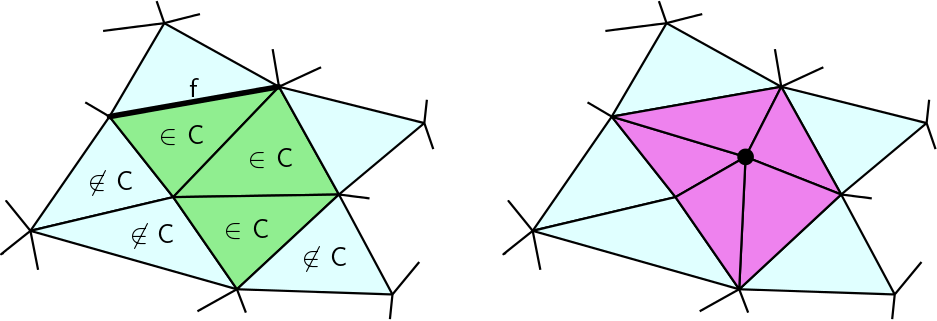Figure 48.4 Insertion in a hole, $$d=2$$

## ◆ insert_increase_dimension()

 Vertex_handle TriangulationDataStructure::insert_increase_dimension ( Vertex_handle star )

Transforms a triangulation of the sphere $$\mathbb{S}^d$$ into the triangulation of the sphere $$\mathbb{S}^{d+1}$$ by adding a new vertex v.

v is used to triangulate one of the two half-spheres of $$\mathbb{S}^{d+1}$$ ( $$v$$ is added as $$(d+2)^{th}$$ vertex to all full cells) and star is used to triangulate the other half-sphere (all full cells that do not already have star as vertex are duplicated, and star replaces v in these full cells). The indexing of the vertices in the full cell is such that, if f was a full cell of maximal dimension in the initial complex, then (f,v), in this order, is the corresponding full cell in the updated triangulation. A handle to v is returned (see Figure Figure 48.5).

Precondition
If the current dimension is -2 (empty triangulation), then star can be omitted (it is ignored), otherwise the current dimension must be strictly less than the maximal dimension and star must be a vertex of tds.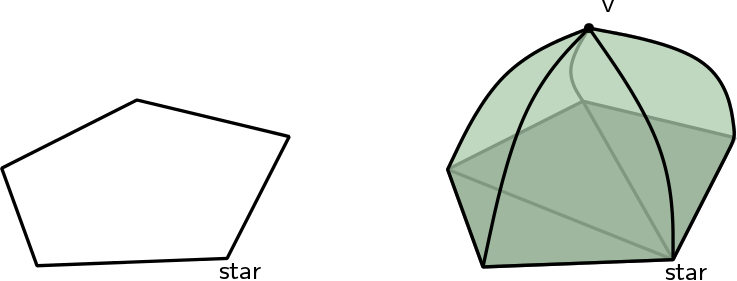Figure 48.5 Insertion, increasing the dimension from $$d=1$$ to $$d=2$$

## ◆ is_valid()

 bool TriangulationDataStructure::is_valid ( bool verbose = false ) const

This is a function for debugging purpose.

Debugging Support

Partially checks whether tds is indeed a triangulation.

It must at least

• check the validity of the vertices and full cells of tds by calling their respective is_valid method.
• check that each full cell has no duplicate vertices and has as many neighbors as its number of facets (current_dimension()+1).
• check that each full cell share exactly tds.current_dimension() vertices with each of its neighbor.

When verbose is set to true, messages are printed to give a precise indication on the kind of invalidity encountered.

Returns true if all the tests pass, false if any test fails. See the documentation for the models of this concept to see the additional (if any) validity checks that they implement.

## ◆ maximal_dimension()

 int TriangulationDataStructure::maximal_dimension ( ) const

Returns the maximal dimension of the full dimensional cells that can be stored in the triangulation tds.

Postcondition
the returned value is positive.

## ◆ mirror_index()

 int TriangulationDataStructure::mirror_index ( Full_cell_handle c, int i ) const

Returns the index of c as a neighbor of its i-th neighbor.

Precondition
$$0 \leq i \leq$$tds.current_dimension() and c!=Full_cell_handle().

## ◆ mirror_vertex()

 Vertex_handle TriangulationDataStructure::mirror_vertex ( Full_cell_handle c, int i ) const

Returns the vertex of the i-th neighbor of c that is not vertex of c.

Precondition
$$0 \leq i \leq$$tds.current_dimension() and c!=Full_cell_handle().

## ◆ neighbor()

 Full_cell_handle TriangulationDataStructure::neighbor ( Full_cell_handle c, int i ) const

Returns a Full_cell_handle pointing to the Full_cell opposite to the i-th vertex of c.

Precondition
$$0 \leq i \leq$$tds.current_dimension() and c != Full_cell_handle()

## ◆ new_full_cell()

 Full_cell_handle TriangulationDataStructure::new_full_cell ( )

Adds a new full cell to tds and returns a handle to it.

The new full cell has no vertices and no neighbors yet.

## ◆ new_vertex()

 Vertex_handle TriangulationDataStructure::new_vertex ( )

Adds a new vertex to tds and returns a handle to it.

The new vertex has no associated full cell nor index yet.

## ◆ operator>>()

 std::istream& TriangulationDataStructure::operator>> ( std::istream & is, TriangulationDataStructure & tds )

Reads a combinatorial triangulation from is and assigns it to tds.

Precondition
The dimension of the input complex must be less than or equal to tds.maximal_dimension().

## ◆ remove_decrease_dimension()

 void TriangulationDataStructure::remove_decrease_dimension ( Vertex_handle v, Vertex_handle star )

This method does exactly the opposite of insert_increase_dimension(): v is removed, full cells not containing star are removed, full cells containing star but not v lose vertex star, full cells containing star and v lose vertex v (see Figure Figure 48.5).

Precondition
All cells contain either star or v. Edge star-v exists in the triangulation and current_dimension() != -2.

## ◆ set_current_dimension()

 void TriangulationDataStructure::set_current_dimension ( int d )

Forces the current dimension of the complex to d.

Precondition
$$-2 \leq d \leq$$maximal_dimension().

## ◆ set_neighbors()

 void TriangulationDataStructure::set_neighbors ( Full_cell_handle ci, int i, Full_cell_handle cj, int j )

Sets the neighbor opposite to vertex i of Full_cell ci to cj.

Sets the neighbor opposite to vertex j of Full_cell cj to ci.

## ◆ star()

template<typename OutputIterator >
 OutputIterator TriangulationDataStructure::star ( const Face & f, OutputIterator out ) const

Inserts in out all the full cells that share at least one vertex with the Face f.

Returns the output iterator.

Precondition
f.full_cell()!=Full_cell_handle().

## ◆ vertex()

 Vertex_handle TriangulationDataStructure::vertex ( Full_cell_handle c, const int i ) const

Returns a handle to the i-th Vertex of the Full_cell c.

Precondition
$$0 \leq i \leq$$tds.current_dimension() and c!=Full_cell_handle().

## ◆ vertices_begin()

 Vertex_iterator TriangulationDataStructure::vertices_begin ( )

Iterator to the first vertex of tds.

User has no control on the order.